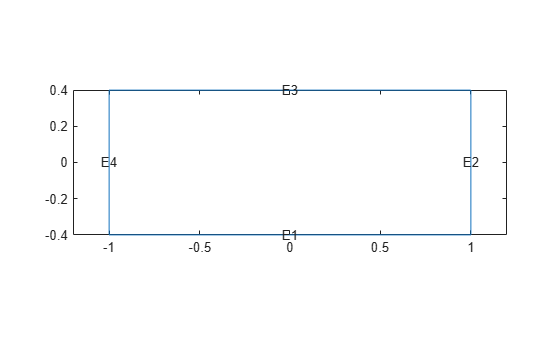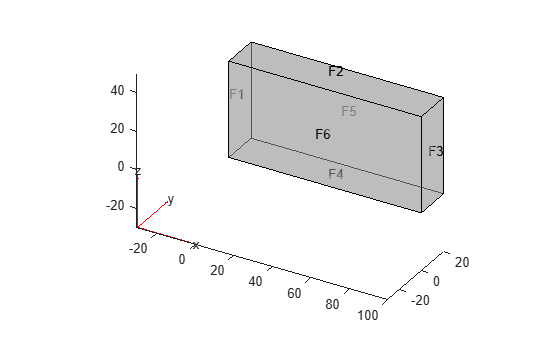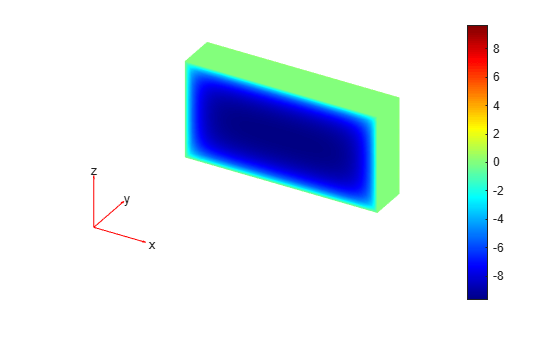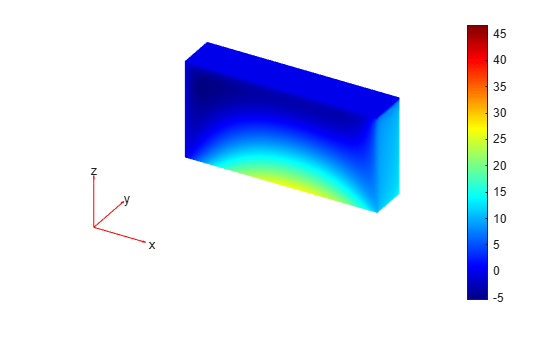Main Content

# applyBoundaryCondition

Add boundary condition to `PDEModel` container

## Syntax

``applyBoundaryCondition(model,'dirichlet',RegionType,RegionID,Name,Value)``
``applyBoundaryCondition(model,'neumann',RegionType,RegionID,Name,Value)``
``applyBoundaryCondition(model,'mixed',RegionType,RegionID,Name,Value)``
``bc = applyBoundaryCondition(___)``

## Description

example

````applyBoundaryCondition(model,'dirichlet',RegionType,RegionID,Name,Value)` adds a Dirichlet boundary condition to `model`. The boundary condition applies to boundary regions of type `RegionType` with ID numbers in `RegionID`, and with arguments `r`, `h`, `u`, `EquationIndex` specified in the `Name,Value` pairs. For Dirichlet boundary conditions, specify either both arguments `r` and `h`, or the argument `u`. When specifying `u`, you can also use `EquationIndex`.```

example

````applyBoundaryCondition(model,'neumann',RegionType,RegionID,Name,Value)` adds a Neumann boundary condition to `model`. The boundary condition applies to boundary regions of type `RegionType` with ID numbers in `RegionID`, and with values `g` and `q` specified in the `Name,Value` pairs.```

example

````applyBoundaryCondition(model,'mixed',RegionType,RegionID,Name,Value)` adds an individual boundary condition for each equation in a system of PDEs. The boundary condition applies to boundary regions of type `RegionType` with ID numbers in `RegionID`, and with values specified in the `Name,Value` pairs. For mixed boundary conditions, you can use `Name,Value` pairs from both Dirichlet and Neumann boundary conditions as needed.```
````bc = applyBoundaryCondition(___)` returns the boundary condition object.```

## Examples

collapse all

Create a PDE model and geometry.

```model = createpde(1); R1 = [3,4,-1,1,1,-1,-.4,-.4,.4,.4]'; g = decsg(R1); geometryFromEdges(model,g);```

View the edge labels.

```pdegplot(model,'EdgeLabels','on') xlim([-1.2,1.2]) axis equal```Apply zero Dirichlet condition on the edge 1.

`applyBoundaryCondition(model,'dirichlet','Edge',1,'u',0);`

On other edges, apply Dirichlet condition `h*u = r`, where `h = 1` and `r = 1`.

`applyBoundaryCondition(model,'dirichlet','Edge',2:4,'r',1,'h',1);`

Create a PDE model and geometry.

```model = createpde(2); R1 = [3,4,-1,1,1,-1,-.4,-.4,.4,.4]'; g = decsg(R1); geometryFromEdges(model,g);```

View the edge labels.

```pdegplot(model,'EdgeLabels','on') xlim([-1.2,1.2]) axis equal```Apply the following Neumann boundary conditions on the edge 4.

`applyBoundaryCondition(model,'neumann','Edge',4,'g',[0;.123],'q',[0;0;0;0]);`

Apply both types of boundary conditions to a scalar problem. First, create a PDE model and import a simple block geometry.

```model = createpde; importGeometry(model,'Block.stl');```

View the face labels.

`pdegplot(model,'FaceLabels','on','FaceAlpha',0.5)`Set zero Dirichlet conditions on the narrow faces, which are labeled 1 through 4.

`applyBoundaryCondition(model,'dirichlet','Face',1:4,'u',0);`

Set Neumann boundary conditions with opposite signs on faces 5 and 6.

```applyBoundaryCondition(model,'neumann','Face',5,'g',1); applyBoundaryCondition(model,'neumann','Face',6,'g',-1);```

Solve an elliptic PDE with these boundary conditions, and plot the result.

```specifyCoefficients(model,'m',0,'d',0,'c',1,'a',0,'f',0); generateMesh(model); results = solvepde(model); u = results.NodalSolution; pdeplot3D(model,'ColorMapData',u)```Create a PDE model and import a simple block geometry.

```model = createpde(3); importGeometry(model,'Block.stl');```

View the face labels.

`pdegplot(model,'FaceLabels','on','FaceAlpha',0.5)`Set zero Dirichlet conditions on faces 1 and 2.

`applyBoundaryCondition(model,'dirichlet','Face',1:2,'u',[0,0,0]);`

Set Neumann boundary conditions with opposite signs on faces 4, 5, and 6.

```applyBoundaryCondition(model,'neumann','Face',4:5,'g',[1;1;1]); applyBoundaryCondition(model,'neumann','Face',6,'g',[-1;-1;-1]);```

For face 3, apply generalized Neumann boundary condition for the first equation and Dirichlet boundary conditions for the second and third equations.

```h = [0 0 0;0 1 0;0 0 1]; r = [0;3;3]; q = [1 0 0;0 0 0;0 0 0]; g = [10;0;0]; applyBoundaryCondition(model,'mixed','Face',3,'h',h,'r',r,'g',g,'q',q);```

Solve an elliptic PDE with these boundary conditions, and plot the result.

```specifyCoefficients(model,'m',0,'d',0,'c',1,'a',0,'f',[0;0;0]); generateMesh(model); results = solvepde(model); u = results.NodalSolution; pdeplot3D(model,'ColorMapData',u(:,1))```## Input Arguments

collapse all

PDE model, specified as a `PDEModel` object.

Example: `model = createpde`

Geometric region type, specified as `'Face'` for 3-D geometry or `'Edge'` for 2-D geometry.

Example: `applyBoundaryCondition(model,'dirichlet','Face',3,'u',0)`

Data Types: `char` | `string`

Geometric region ID, specified as a vector of positive integers. Find the region IDs using `pdegplot` with the `'FaceLabels'` (3-D) or `'EdgeLabels'` (2-D) value set to `'on'`.

Example: `applyBoundaryCondition(model,'dirichlet','Face',3:6,'u',0)`

Data Types: `double`

### Name-Value Pair Arguments

Example: `applyBoundaryCondition(model,'dirichlet','Face',1:4,'u',0)`

Dirichlet condition `h*u = r`, specified as a vector with N elements or a function handle. N is the number of PDEs in the system. For the syntax of the function handle form of `r`, see Nonconstant Boundary Conditions.

Example: `'r',[0;4;-1]`

Data Types: `double` | `function_handle`
Complex Number Support: Yes

Dirichlet condition `h*u = r`, specified as an N-by-N matrix, a vector with N^2 elements, or a function handle. N is the number of PDEs in the system. For the syntax of the function handle form of `h`, see Nonconstant Boundary Conditions.

Example: `'h',[2,1;1,2]`

Data Types: `double` | `function_handle`
Complex Number Support: Yes

Generalized Neumann condition `n·(c×``u) + qu = g`, specified as a vector with N elements or a function handle. N is the number of PDEs in the system. For scalar PDEs, the generalized Neumann condition is `n·(c````u) + qu = g```. For the syntax of the function handle form of `g`, see Nonconstant Boundary Conditions.

Example: `'g',[3;2;-1]`

Data Types: `double` | `function_handle`
Complex Number Support: Yes

Generalized Neumann condition `n·(c×``u) + qu = g`, specified as an N-by-N matrix, a vector with N`^2` elements, or a function handle. N is the number of PDEs in the system. For the syntax of the function handle form of `q`, see Nonconstant Boundary Conditions.

Example: `'q',eye(3)`

Data Types: `double` | `function_handle`
Complex Number Support: Yes

Dirichlet conditions, specified as a vector of up to N elements or as a function handle. If `u` has less than N elements, then you must also use `EquationIndex`. The `u` and `EquationIndex` arguments must have the same length. If `u` has N elements, then specifying `EquationIndex` is optional.

For the syntax of the function handle form of `u`, see Nonconstant Boundary Conditions.

Example: `applyBoundaryCondition(model,'dirichlet','Face',[2,4,11],'u',0)`

Data Types: `double`
Complex Number Support: Yes

Index of the known `u` components, specified as a vector of integers with entries from `1` to N. `EquationIndex` and `u` must have the same length.

When using `EquationIndex` to specify Dirichlet boundary conditions for a subset of components, use the `mixed` argument instead of `dirichlet`. The remaining components satisfy the default Neumann boundary condition with the zero values for `'g'` and `'q'`.

Example: `applyBoundaryCondition(model,'mixed','Face',[2,4,11],'u',[3,-1],'EquationIndex',[2,3])`

Data Types: `double`

Vectorized function evaluation, specified as `'on'` or `'off'`. This evaluation applies when you pass a function handle as an argument. To save time in function handle evaluation, specify `'on'`, assuming that your function handle computes in a vectorized fashion. See Vectorization. For details of this evaluation, see Nonconstant Boundary Conditions.

Example: `applyBoundaryCondition(model,'dirichlet','Face',[2,4,11],'u',@ucalculator,'Vectorized','on')`

Data Types: `char` | `string`

## Output Arguments

collapse all

Boundary condition, returned as a BoundaryCondition Properties object. The `model` object contains a vector of `BoundaryCondition` objects. `bc` is the last element of this vector.

## Tips

• When there are multiple boundary condition assignments to the same geometric region, the toolbox uses the last applied setting.

• To avoid assigning boundary conditions to a wrong region, ensure that you are using the correct geometric region IDs by plotting and visually inspecting the geometry.

• If you do not specify a boundary condition for an edge or face, the default is the Neumann boundary condition with the zero values for `'g'` and `'q'`.

## See Also

### Topics

Introduced in R2015a

## SupportGet trial now